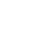Online Math Quiz Sets of 10 Questions each. It will take around 5 minutes of your time to complete a set of questions. Solving this math test quiz will help you to know how much you know about basic math functions. You can share these questions with your friends over and have some fun.

15
Created on Byadmin

Math Quiz 1

Math Quiz

1 / 10

Find the sum of 111 + 222 + 333

2 / 10

Simplify: 240 ÷ (9 + 3 x 7) - 5

3 / 10

Solve: 35 + 7 ÷ 7

4 / 10

Simplify: 150 ÷ (6 + 3 x 8) - 5

5 / 10

Simplify : 3 + 6 x (5 + 4) ÷ 3 - 7

6 / 10

Solve: 365 – (128 ÷ 4)

7 / 10

Subtract 264 from 1094

8 / 10

Find the product of 78 × 3

9 / 10

Select the Answer for 110 ÷ 10

10 / 10

Simplify: 26 + 32 - 12